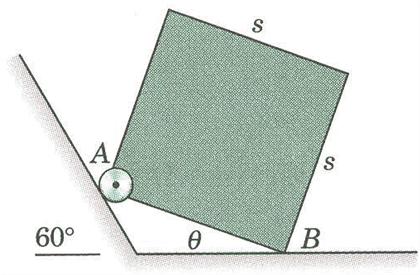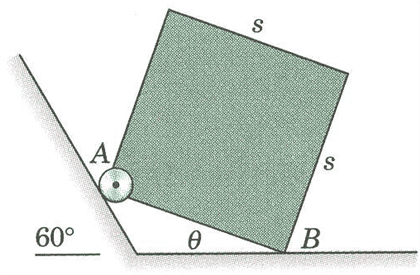# Statics Equilibrium: Normal Force and Static Friction

• jwp0015

#### jwp0015

1. The homogeneous square body is positioned as shown. If the coefficient of static friction at B is 0.40, determine the critical value of the angle theta below which slipping will occur. Neglect friction at A.

The image:2. NA is the Normal force at A, which is perpindicular to the 60° incline.
NB is the Normal force at B, which is in the positive y direction.
W=mg which is the weight in the negative y direction.
ƩFx=0
ƩFy=0
ƩMB=0 (the moment about B eliminates the unknowns NB and Ff.

3. I have three pages of handwritten work. I started with the Moment about B, and took the moment arm from B to the center of mass (s/2)((sin∅-cos∅)i + (sin∅+cos∅)j. W is simply -Wj. I took the moment arm for the NA force to be s(-cos∅i + sin∅j). NA=NA(cos(30)i + sin(30)j). Putting all of those together into the ƩMB= r1 x W + r2 x NA = 0.

I took the cross products and then summed the y forces and x forces. I have not been able to find equations to set equal to each other or substitue into each other to end up with an answer of (some tangent function of ∅) = (some number)

The answer to the problem is 20.7, but I have had no luck getting there. Thanks for the help ahead of time.

1. The homogeneous square body is positioned as shown. If the coefficient of static friction at B is 0.40, determine the critical value of the angle theta below which slipping will occur. Neglect friction at A.

The image:2. NA is the Normal force at A, which is perpindicular to the 60° incline.
NB is the Normal force at B, which is in the positive y direction.
W=mg which is the weight in the negative y direction.
ƩFx=0
ƩFy=0
ƩMB=0 (the moment about B eliminates the unknowns NB and Ff.

3. I have three pages of handwritten work. I started with the Moment about B, and took the moment arm from B to the center of mass (s/2)((sin∅-cos∅)i + (sin∅+cos∅)j. W is simply -Wj. I took the moment arm for the NA force to be s(-cos∅i + sin∅j). NA=NA(cos(30)i + sin(30)j). Putting all of those together into the ƩMB= r1 x W + r2 x NA = 0.

I took the cross products and then summed the y forces and x forces. I have not been able to find equations to set equal to each other or substitue into each other to end up with an answer of (some tangent function of ∅) = (some number)

The answer to the problem is 20.7, but I have had no luck getting there. Thanks for the help ahead of time.

What you have shown from your work looks correct.
Show your further work in detail so we can see what went wrong.

ehild